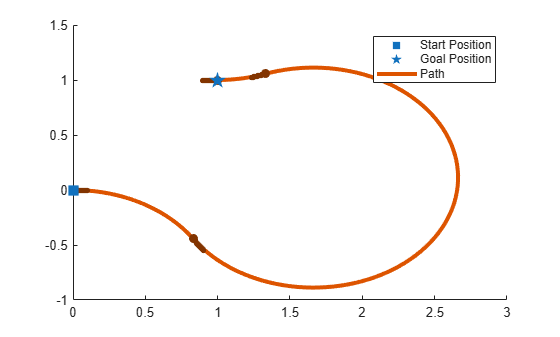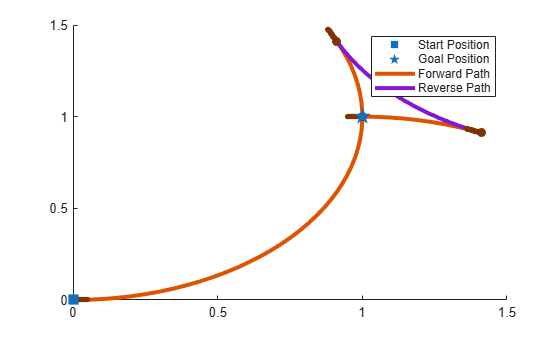Main Content

# connect

Connect poses for given connection type

## Syntax

``[pathSegments,pathCosts] = connect(connectionObj,start,goal)``
``[pathSegments,pathCosts] = connect(connectionObj,start,goal,'PathSegments','all')``

## Description

example

````[pathSegments,pathCosts] = connect(connectionObj,start,goal)` connects the start and goal poses using the specified `dubinsConnection` object. The path segment object with the lowest cost is returned.```
````[pathSegments,pathCosts] = connect(connectionObj,start,goal,'PathSegments','all')` returns all possible path segments as a cell array with their associated costs.```

## Examples

collapse all

Create a `dubinsConnection` object.

`dubConnObj = dubinsConnection;`

Define start and goal poses as `[x y theta]` vectors.

```startPose = [0 0 0]; goalPose = [1 1 pi];```

Calculate a valid path segment to connect the poses.

`[pathSegObj, pathCosts] = connect(dubConnObj,startPose,goalPose);`

Show the generated path.

`show(pathSegObj{1})`Create a `reedsSheppConnection` object.

`reedsConnObj = reedsSheppConnection;`

Define start and goal poses as `[x y theta]` vectors.

```startPose = [0 0 0]; goalPose = [1 1 pi];```

Calculate a valid path segment to connect the poses.

`[pathSegObj,pathCosts] = connect(reedsConnObj,startPose,goalPose);`

Show the generated path.

`show(pathSegObj{1})`## Input Arguments

collapse all

Path connection type, specified as a `dubinsConnection` or `reedsSheppConnection` object. This object defines the parameters of the connection, including the minimum turning radius of the robot and the valid motion types.

This property is read-only.

Initial pose of the robot at the start of the path segment, specified as an [x, y, Θ] vector or matrix. Each row of the matrix corresponds to a different start pose.

x and y are in meters. Θ is in radians.

The `connect` function supports:

• Singular start pose with singular goal pose.

• Multiple start pose with singular goal pose.

• Singular start pose with multiple goal pose.

• Multiple start pose with multiple goal pose.

The output `pathSegments` cell array size reflects the singular or multiple pose options.

This property is read-only.

Goal pose of the robot at the end of the path segment, specified as an [x, y, Θ] vector or matrix. Each row of the matrix corresponds to a different goal pose.

x and y are in meters. Θ is in radians.

The `connect` function supports:

• Singular start pose with singular goal pose.

• Multiple start pose with singular goal pose.

• Singular start pose with multiple goal pose.

• Multiple start pose with multiple goal pose.

The output `pathSegments` cell array size reflects the singular or multiple pose options.

## Output Arguments

collapse all

Path segments, specified as a cell array of objects. The type of object depends on the input `connectionObj`. The size of the cell array depends on whether you use singular or multiple `start` and `goal` poses. By default, the function returns the path with the lowest cost for each `start` and `goal` pose. When call `connect` using the `'PathSegments','all'` name-value pair, the cell array contains all valid path segments between the specified `start` and `goal` poses.

Cost of path segments, specified as a positive numeric scalar, vector, or matrix. Each element of the cost vector or matrix corresponds to a path segment in `pathSegment`. By default, the function returns the path with the lowest cost for each start and goal pose.

Example: `[7.6484,7.5122]`

## Extended Capabilities

### C/C++ Code GenerationGenerate C and C++ code using MATLAB® Coder™.

Introduced in R2019b